## Complement Set

Given a setwith a subset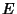, the complement ofis defined as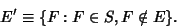(1)

If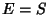, then(2)

where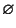is the Empty Set. Given a single Set, the second Probability Axiom gives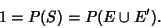(3)

Using the fact that,(4)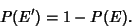(5)

This demonstrates that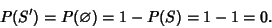(6)

Given two Sets,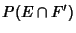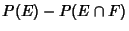(7)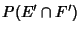(8)